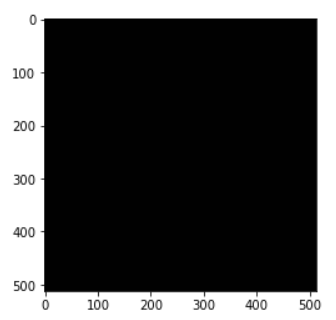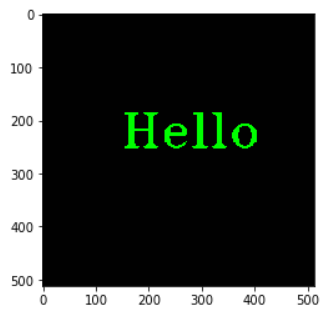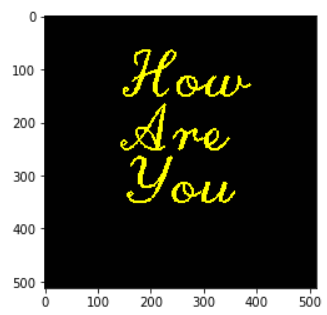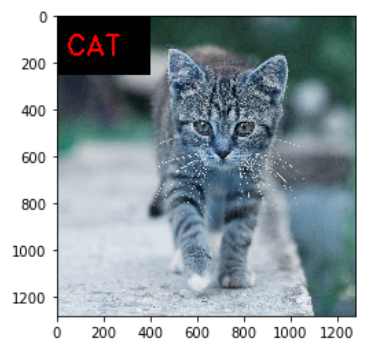# Put Text on Image in OpenCV Python using cv2.putText() with Examples## Introduction

In this article, we will understand how to put text on images in OpenCV using cv2.putText() function. There are many scenarios where you may have to write text on an image either to annotate or maybe just for doing debugging, etc. Here we will explain to you cv2.putText() syntax along with few examples so that it can be understood clearly, especially for beginners.

## Put Text on Image in OpenCV Python : cv2.putText()

We can put text on images in OpenCV python quite easily by using cv2.putText() function. The syntax of this function is shown below –

### Syntax

cv2.putText(img, text, org, fontFace, fontScale, color, thickness)

• img – It is the image on which the text has to be written.
• text – It is the text that needs to be put on the image
• org – Bottom-left corner of the text string in the image.
• fontFace – The font of the text. See the font types available in OpenCV here.
• fontScale – This value scales the size of the text by multiplying its base size.
• color – The color of the text.
• thickness – The thickness of the line of text.

## Examples of cv2.putText() in Python OpenCV

### Import Required Libraries

Before starting the examples let us first import the required libraries as shown below –

In :

```import cv2
import numpy as np
import matplotlib.pyplot as plt

%matplotlib inline```

### Utility Function to Create Empty Image

We will write a utility function that helps to create an empty image of the size 512×512 and 3 color channels. We will use this blank image to write text onto it.

In :

```def generate_empty_image():

return np.ones(shape=(512,512,3), dtype=np.int16)```

Let us see this function in action by calling it below.

In :

```sample_img = generate_empty_image()
plt.imshow(sample_img)```

Out:

<matplotlib.image.AxesImage at 0x1e14d0dc9b0>### Example – 1: Put Text on Image with cv2.putText()

In this first example, we will write a simple text on the blank image whose color is green, font is FONT_HERSHEY_TRIPLEX, font scale is 3 and thickness is 3.

In :

```img = generate_empty_image()

cv2.putText(img=img, text='Hello', org=(150, 250), fontFace=cv2.FONT_HERSHEY_TRIPLEX, fontScale=3, color=(0, 255, 0),thickness=3)

plt.imshow(img)```

Out:

<matplotlib.image.AxesImage at 0x1531e80ec50>### Example – 2: Put Text on Multiple Lines in cv2.putText()

OpenCV putText() function does not support writing text on multiple lines out of the box. We have to use certain workarounds to achieve this.

In the below example, we split the text based on the newline character and then write each of the lines on the image in a loop.

In :

```img1 = generate_empty_image()

text = "How\nAre\nYou"

y_start = 150

y_increment = 100

for i, line in enumerate(text.split('\n')):
y = y_start + i*y_increment
cv2.putText(img=img1, text=line, org=(150, y), fontFace=cv2.FONT_HERSHEY_SCRIPT_COMPLEX, fontScale=4, color=(255,255,0),
thickness=3)

plt.imshow(img1)```

Out:

<matplotlib.image.AxesImage at 0x15325aeecf8>### Example – 3: Put Text with Background Color on Image in cv2.putText()

OpenCV cv2.putText() does not have any built-in capabilities to have a background color for the text. But there is a workaround for achieving this effect.

We can create a small rectangle of the desired color on the image and put text on the rectangle.

In the below example, we have created a black color rectangle and have written the text inside it by calculating the various coordinates.

In :

```img3 = cv2.imread('cat.jpg')

x,y,w,h = 0,0,400,250

# Create background rectangle with color
cv2.rectangle(img3, (x,x), (x + w, y + h), (0,0,0), -1)

cv2.putText(img=img3, text="CAT",org=(x + int(w/10),y + int(h/1.5)), fontFace=cv2.FONT_HERSHEY_DUPLEX, fontScale=4, color=(255,0,0), thickness=7)

plt.imshow(img3)```

Out:

<matplotlib.image.AxesImage at 0x1532cf0b860>## Conclusion

Hope you found this quick tutorial on writing text on images in OpenCV quite helpful. We covered the syntax of the cv2.putText() function along with various examples for a better understanding of beginners.

References: